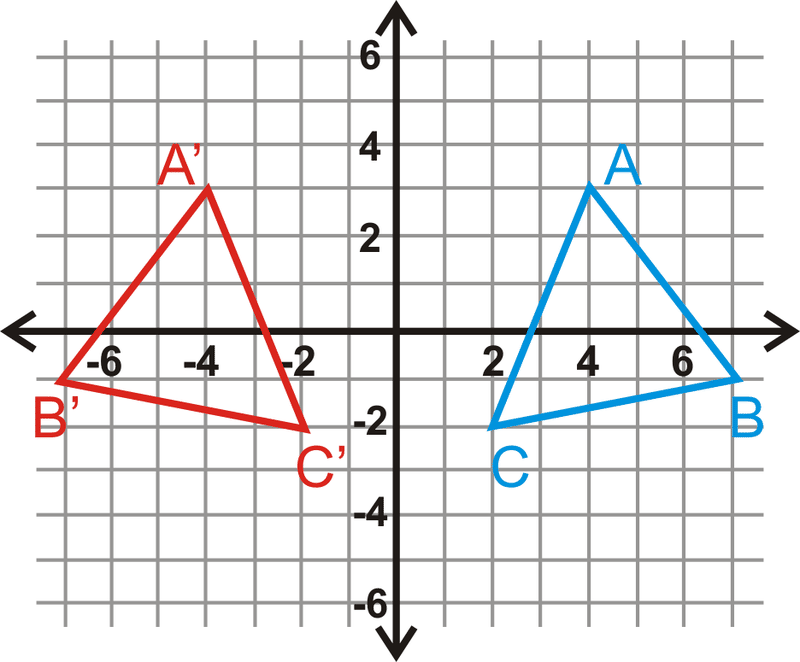Breaking News
Home / SSC / SSC CGL MAINS – REFLECTION in Coordinate Geometry Study Material

# SSC CGL MAINS – REFLECTION in Coordinate Geometry Study MaterialSSC CGL MAINS

REFLECTION in Coordinate Geometry Study Material

In 2017, SSC asked reflection in coordinate geometry questions in CGL 17 PRE & MTS 16 Online Exams. So, its quite probable that SSC will ask reflection in coordinate geometry questions in CGL 17 Mains.

Some insights on Reflection in Coordinate Geometry TopicCommon Reflections

• Reflection over y=x: if (x,y) is reflected over the line y=x, then the image is (y,x) (y,x).
• Reflection over y=b: if (x,y) (x,y)is reflected over the horizontal line y=b, then the image is (x,2b-y)(x,2by).
• Reflection over  x=a: if (x,y)  (x,y)is reflected over the vertical line  x=a x=a, then the image is (2a-x, y)y).
• Reflection over the  x-axis: if (x,y)  is reflected over the  x-x−axis, then the image is (x,-y) (x,−y).
• Reflection over the y-axis:If ((x,y)  x,y) is reflected over the  y-−axis, then the image is (-x,y) (−x,y).
• Reflection over y=-xy=−x:If  (x,y)  is reflected over the line y=-x y=−x, then the image is (-y,-x).
India's No. 1 SSC Test Series for SSC CGL/CPO/CHSL - Click Here

## Daily Dose by SSCtube – One Word Substitution (12-03-2019)

Daily Dose by SSCtube (One Word Substitution) Attempt SSC CGL 18/CPO 18/RRB ALP/RRB GROUP D …

## Daily Dose by SSCtube – Para Jumble (PQRS) (11-03-2019)

Daily Dose by SSCtube (Para Jumble (PQRS)) Attempt SSC CGL 18/CPO 18/RRB ALP/RRB GROUP D …

## Daily Dose by SSCtube – Ratio and Proportion (4-3-2019)

Daily Dose by SSCtube (Ratio and Proportion) Attempt SSC CGL 18/CPO 18/RRB ALP/RRB GROUP D …